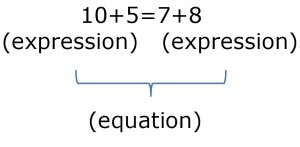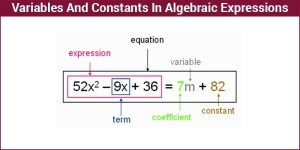Option Training Institute FZ LLC G 37 & G 34, Block 13, Al Sufouh Road Knowledge, Village Dubai PO Box : 501703

# FUNDAMENTAL CONCEPTS AND TECHNIQUES IN ALGEBRAIC EXPRESSIONSEQUATION:

A mathematical statement in which two expressions are equal to one another and set on either side of an equal sign:VARIABLE:

A symbol (often a lower-case or upper-case letter) that represents one or more numbers. For instance, in the equation a+b=c+5, a, b and c are variables.

When an equation includes a variable, the numerical value of that variable which makes the equation true (makes the two sides equal to each other) is called the solution to the equation. An equation may have more than one solution, or it may have no solutions.

CONSTANT:

A fixed value. In Algebra, a constant is a number on its own, or sometimes a letter such as a, b or c to stand for a fixed number.

Example: in “x + 5 = 9”, 5 and 9 are constants.

COEFFICIENT:

A Coefficient is a number used to multiply a variable (4x means 4 times x, so 4 is a coefficient).

Variables on their own (without a number next to them) actually have a coefficient of 1 (x is really 1x).INEQUALITIES:

An inequality shows that two mathematical expressions are NOT equal to one another.

Inequalities are used to show that one quantity is greater than (>), less than (<), greater than or equal (≥), less than or equal(≤), or not equal (≠) to another quantity.

Example:

10+x≥ 9 (means “any quantity that is ten greater than x is greater than or equal to nine”.)

Solving inequalities is much like solving equations, but with one very important exception “when solving inequalities, reverse the direction of the inequality sign whenever you multiply or divide the inequality by a negative number”.

## ALGEBRAIC WORD PROBLEMS

When you encounter an algebraic word problem, convert the sentence into algebraic expressions by converting words that indicate relations and operations into symbols.

Examples include:

• “is” means “=”
• “more than”, “the sum of”, and “increased by ” mean “+”
• “less than” and “the difference” mean “-“
• “the product of “ means “×”

Always begin by examining the question for structures and expressions that consist of more than just a single variable or number that will help manipulate the equations. Most questions will contain such a structure, and difficult questions may require you to recognize a question’s structure to answer the question at all.

An Important strategy to approach this type of question uses the property of equality to isolate the variable on one side of the equation and solve it. Confirm the solution by plugging the value back into the original equation.

When working with an equation or inequality with more than one variable, it will often be necessary to solve for one variable. Certain questions will ask you to do only this. To solve for a given variable, transform the equation or inequality so that the variable is isolated on one side of the equation. This variable is then said to be expressed in terms of the other variable(s).

5 STEPS TO FOLLOW:

1. Read the problem carefully and determine what values you are trying to find.

2. Assign variables to unknown values if appropriate; Make a sketch.

3. Write an equation or equations that represent(s) the relationship among values.

4. Solve the equation or equations.

5. Check any solutions in the context of the problem.

At Option Training Institute, Dubai, Training for tests such as the SAT and ACT is a planned exercise that aims at making the learning of math an enjoyable and productive engagement.